profit per t2 item

Forum rules
This forum can be viewed by the public.

Production Managerprofit per t2 item

Hey,
Is the math here more or less correct or did I forget some aspects?
SRP Officer | Graduate | Killboard Staff | Production Manager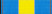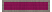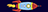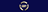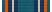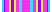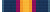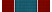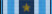MemberRe: profit per t2 item

I don't do T2 production but some thoughts on your latex:
Don't write "SCI = System Cost Index" but "$SCI$ = System Cost Index". This way "SCI" is treaded the same way like later in your formulas. Further, if you use "SCI" this basically means S times C times I. Use "\mathrm{SCI}" if you really want to name it that way. Better call it alpha or so.
Later you even write stuff out in the formulas. This makes the formulas even less readable. Again use "\mathrm{}" or better use real variables for them.
* is not the mathematical symbol for multication. It is used on a keyboard because it's easy to type. Use "\times" or "\cdot" depending on the style you prefer and context you are in. In this context best chose other variable names and don't use a symbol at all.

On the math:
Let me abbreviate success% with P. Then you have a term (1 + (1-P) / P ) this is the same as 1/P. Which should be the right term. If your probability is 1 you exacly want the price of 1 invention. If it's 1/2 you want the price of 2 inventions and so on.
But that term 1/P should be multiplied with the cost index and the tax too. So just write P into the denominator with the number of runs.

I haven't checked the other formulas in detail.

MemberRe: profit per t2 item

You havn't factored in any structure bonuses which varies structure to structure.MemberRe: profit per t2 item

Not accounting T1 modules production is a mistake, the same mistake if you don't account self-mined minerals.

You have to calculate it with minimum sell-order/max buy-order price and/or with the actual production cost. Which leds to a recurrent calculation down to minerals/moon goo/pi. Factor in your haul time/cost.

Every step should make a little profit in isk or time.MemberRe: profit per t2 item